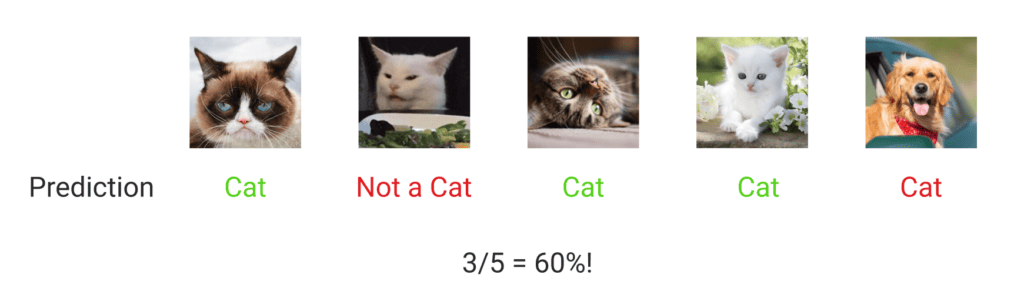## 1. Introduction

When using Machine Learning, we have different metrics that show us to know how well our model is doing. However, these measures can confuse what they mean, how they can be interpreted, or what they are exactly. Knowing this, we could infer more information about our models.

In this tutorial, we’ll focus on loss and accuracy. Both of them are essential values to take into account when training our models.

## 2. Loss

Loss is a value that represents the summation of errors in our model. It measures how well (or bad) our model is doing. If the errors are high, the loss will be high, which means that the model does not do a good job. Otherwise, the lower it is, the better our model works.

To calculate the loss, a loss or cost function is used. There are several different cost functions to use. Each penalizes errors in different ways, and the problem determines which one is better to use. Cross-Entropy and Mean Squared Error are the most commonly used for classification and regression problems, respectively.

But how do we know if the loss is high or low?  Well, it depends on the problem and the cost function being used.

Let’s say we want to predict the color of a pixel, for whatever reason. For simplicity, let’s assume the value of a pixel can go from 0 to 255. We use the Mean Squared Error as our cost function.

In this example, having a loss of 1 would be tiny, while a loss of 100 would be really high:However, whether the loss is high or low is not the most important inference we can learn from it. If we plot loss results over time, we can see whether our model is learning, and how fast.

This is because, in Deep Learning, the loss function is used by the model to learn. The goal of the model is to minimize the value of the loss. This is done by using techniques such as gradient descent, which changes the model parameters using the information of the result of the loss.

Seeing the loss over time can yield interesting findings of our models. If the loss value is not decreasing, but it just oscillates, the model might not be learning at all. However, if it’s decreasing in the training set but not in the validation set (or it decreases but there’s a notable difference), then the model might be overfitting. In other words, it might be overlearning from the training examples, becoming useless in new examples. If that’s the case, it would be interesting to use regularization, use simpler models, or, in Deep Learning, just reduce the learning rate.

In our article about Learning Curves, we can learn more about how to interpret these and other types of model data over time.

## 3. Accuracy

Accuracy is more straightforward. It measures how well our model predicts by comparing the model predictions with the true values in terms of percentage.

For example, let’s say we have a model for image classification that detects whether or not there is a cat in the image. We have 5 test images. If the model is able to predict correctly whether or not there is a cat in 3 of the images, it results in an accuracy of 60%:Now, if we analyze these two measurements together, we can infer more information about how is our model working:

Having a low accuracy but a high loss would mean that the model makes big errors in most of the data. But, if both loss and accuracy are low, it means the model makes small errors in most of the data. However, if they’re both high, it makes big errors in some of the data. Finally, if the accuracy is high and the loss is low, then the model makes small errors on just some of the data, which would be the ideal case.## 4. Conclusion

In this tutorial, we’ve seen what the loss and accuracy metrics are. We’ve seen how to infer information from them, analyzing them on their own and together, and thanks to it being able to know more about how our models work and behave.